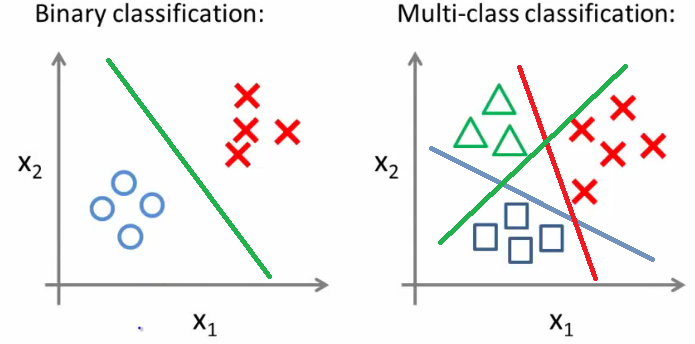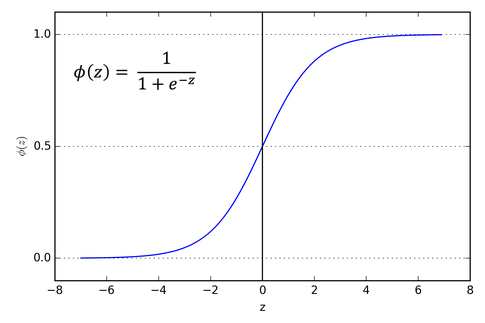Introduction to Logistic Regression

Introduction to Logistic Regression

Introduction to Logistic Regression

Logistic regression is used for binary classification (two class classification) as well as multi-class classification.A straight line may or may not classify the data in all cases.

In some cases, the data needs to be separated by a non-linear curve.

Thus logistic regression uses a Sigmoid or Logistic function, which can take any value and return a value between 0 and 1.

Logistic regression makes use of probability (but its classification algorithm right?). Yes it is.

For eg. We are trying to predict whether the passengers in Titanic survived or not (Based on the famous Titanic problem). We will get the probability values such as 0.5, 0.6,0.3 etc.

Based on the threshold the output is decided to be either 0 or 1.Suppose the threshold is 0.5 then every probability above 0.5 gives output as 1 and less than 0.5 gives output as 0.

By: Mihir Rane